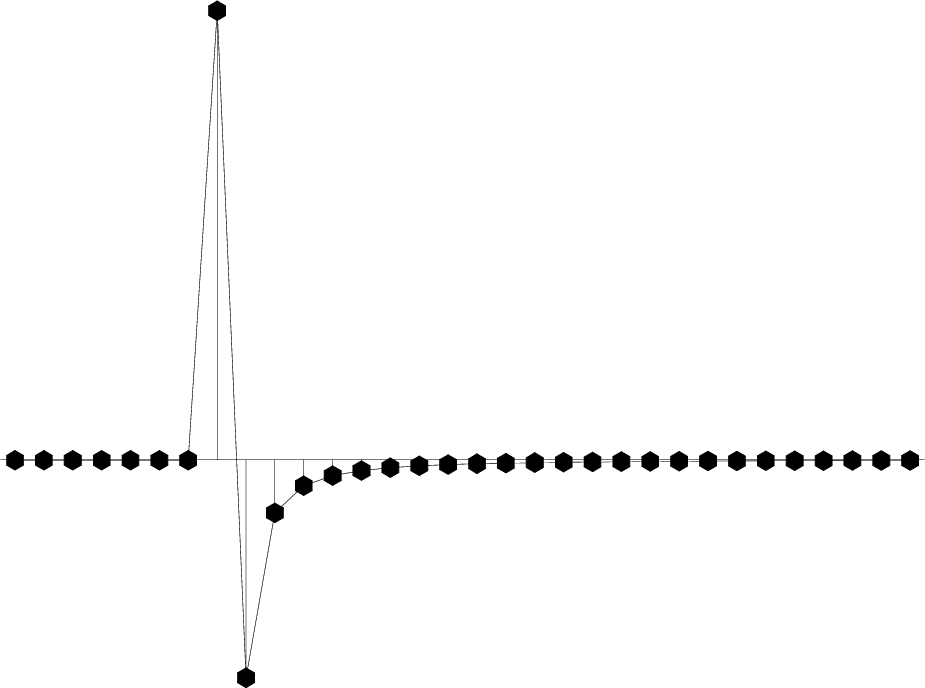sfhalfint implements half-order integration or differentiation, a filtering operation common in 2-D imaging operators such as as slant stacking or Kirchhoff migration.

By default, sfhalfint performs half-order integration. To apply half-order differentiation, use inv=y. To apply the adjoint operator, use adj=y.

Theoretically, half-order integration and differiation correspond to division by $(i\omega)^{1/2}$. For stability, $i\omega$ is replaced in practice by $1-\rho\,Z$ when doing differentiation and by $\frac{1}{2}\,\frac{1-\rho\,Z}{1+\rho\,Z}$ when doing integration. Here $Z=e^{i\omega\,\Delta t}$. As explained by Jon Claerbout, this approximation attenuates high frequencies and assures a causal impulse response. The value of the $\rho$ parameter is controlled by rho=.

The following plot from bei/ft1/hankel shows the impulse response of half-order differentiation (also known as the “rho filter”)